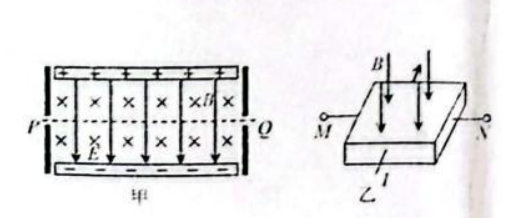$\text{A.}$ 图甲中, 电子以速度大小 $v=\frac{E}{B}$ 从 $Q$ 端射入, 可沿直线运动从 $P$ 点射出 $\text{B.}$ 图甲中, 电子以速度大小 $v>\frac{E}{B}$ 从 $P$ 端射入, 电子向下偏转,轨迹为抛物线 $\text{C.}$ 图乙中, 仅增大电流 $I$, 其他条件不变, $M N$ 之间的霍尔电压将增大 $\text{D.}$ 图乙中, 稳定时霍尔元件 $M$ 侧的电势低于 $N$ 侧的电势

CD